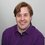# Euclidean algorithm

I found something interesting about solving this question:

If p is the number of pairs of values (x, y) that can make the equation 84x + 140y = 28 true, what is true about p?

I noticed while solving that I could simplify the equation:

84x - 140y = 28

42x – 70y = 14

21x – 35y = 7

3x – 5y = 1

Noting that 3x = 5y + 1 means that 3x must end in 5 + 1 = 6 or 0 + 1 = 1 to allow y to be divisible by 5.

This means 3x should end in either 1 or 6 to be equal to 5y + 1

Finding the following values for x and matching values for y:

3x = 6, 21, 36, 51, 66, 81, 96, 111, 126, 141, 156

5y = 5, 20, 35, 50, 65, 80, 95, 110, 125, 140, 155

Then finding the series of x and y:

X = 2, 7, 12, 17, 22, 27, 32, 37, 42, 47, 52

Y = 1, 4, 7, 10, 13, 16, 19, 22, 25, 28, 31

X seems to be increasing by 5, and Y by 3.

Which means that you can find pairs of x and y by the formulas:

x = 2+5a and y = 1+3a

Leading to a sequence that will satisfy 84x + 140y = 28.

Would this also be more generally true? I also thought it might be an interesting additional question to the quiz.

On another note, this text editor does not seem to like enters in paragraphs. Any way I can fix this?Note by Sytse Durkstra
3 years, 10 months ago

This discussion board is a place to discuss our Daily Challenges and the math and science related to those challenges. Explanations are more than just a solution — they should explain the steps and thinking strategies that you used to obtain the solution. Comments should further the discussion of math and science.

When posting on Brilliant:

• Use the emojis to react to an explanation, whether you're congratulating a job well done , or just really confused .
• Ask specific questions about the challenge or the steps in somebody's explanation. Well-posed questions can add a lot to the discussion, but posting "I don't understand!" doesn't help anyone.
• Try to contribute something new to the discussion, whether it is an extension, generalization or other idea related to the challenge.

MarkdownAppears as
*italics* or _italics_ italics
**bold** or __bold__ bold
- bulleted- list
• bulleted
• list
1. numbered2. list
1. numbered
2. list
Note: you must add a full line of space before and after lists for them to show up correctly
paragraph 1paragraph 2

paragraph 1

paragraph 2

[example link](https://brilliant.org)example link
> This is a quote
This is a quote
    # I indented these lines
# 4 spaces, and now they show
# up as a code block.

print "hello world"
# I indented these lines
# 4 spaces, and now they show
# up as a code block.

print "hello world"
MathAppears as
Remember to wrap math in $$ ... $$ or $ ... $ to ensure proper formatting.
2 \times 3 $2 \times 3$
2^{34} $2^{34}$
a_{i-1} $a_{i-1}$
\frac{2}{3} $\frac{2}{3}$
\sqrt{2} $\sqrt{2}$
\sum_{i=1}^3 $\sum_{i=1}^3$
\sin \theta $\sin \theta$
\boxed{123} $\boxed{123}$

Sort by:

Yes, you just applied Euclidean algorithm.

To enter $\LaTeX$, type like

\ ( ... \ )

but remove the spaces.

- 3 years, 9 months ago